In :
# %load ../../preconfig.py
%matplotlib inline
import matplotlib.pyplot as plt
import seaborn as sns
sns.set(color_codes=True)
plt.rcParams['axes.grid'] = False

#import numpy as np
#import pandas as pd
#import itertools

import logging
logger = logging.getLogger()


# 22 Elementary Graph Algorithms¶

This chapter presents methods for representing a graph and for searching a graph.

### 22.1 Representations of graphs¶

• pros: save memory.

• pros: tell quickly if there is an edge connecting two given vertices.
• $A = A^T$, in some applications, only store half of entries.
In :
plt.figure(figsize=(12,8))

Out:
<matplotlib.image.AxesImage at 0x10a6e8780>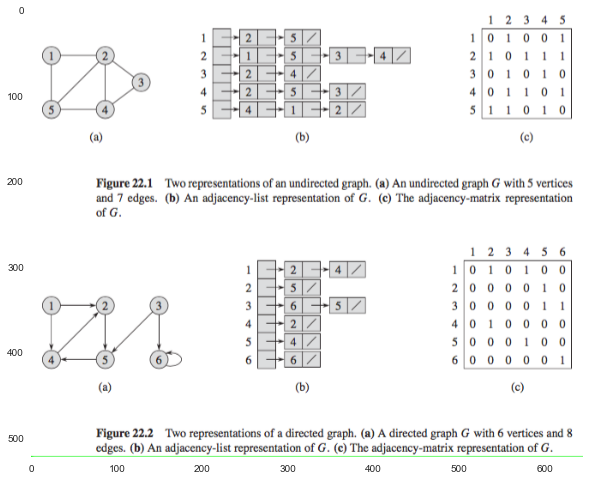In :
# Exercises


Given a graph $G = (V, E)$ and a distinguished source vertex $x$. The breadth-first search computes the distance (smallest number of edges) from $s$ to each reachable vertex.

For any vertex $v$ reachable from $s$, the simle path in the breadth-first tree from $s$ to $v$ corresponds to "shortest path" from $s$ to $v$ in $G$.

In :
plt.imshow(plt.imread('./res/BFS.png'))

Out:
<matplotlib.image.AxesImage at 0x10a748f98>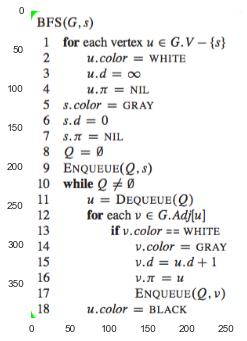In :
plt.figure(figsize=(8,12))

Out:
<matplotlib.image.AxesImage at 0x10ce68978>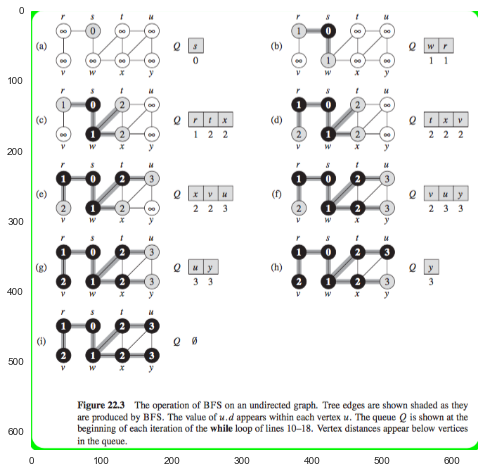In :
# Exercises


Depth-first search explores edges out of the most recently discovered vertex $v$ that still has unexplored edges leaving it.

depth-first search may produce several trees $\to$ we use the predecessor subgraph: $G_{\pi} = (V, E_{\pi})$, where $E_{\pi} = \{ (v.{\pi}, v) : v \in V \text{ and } v.{\pi} \neq \operatorname{NIL} \}$.

1. Each vertex is initially white, is grayed when it is discovered in the search, and is blacked when it is finished.

2. depth-first search also timestamps each vertex.

In :
plt.imshow(plt.imread('./res/DFS.png'))

Out:
<matplotlib.image.AxesImage at 0x1098bd898>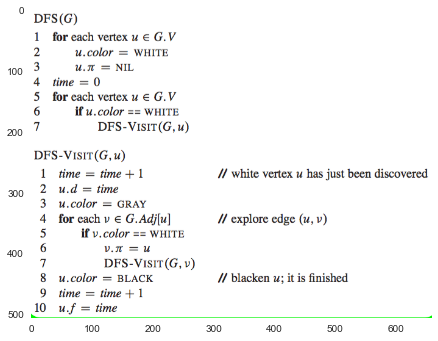In :
plt.figure(figsize=(10,12))

Out:
<matplotlib.image.AxesImage at 0x10bcec630>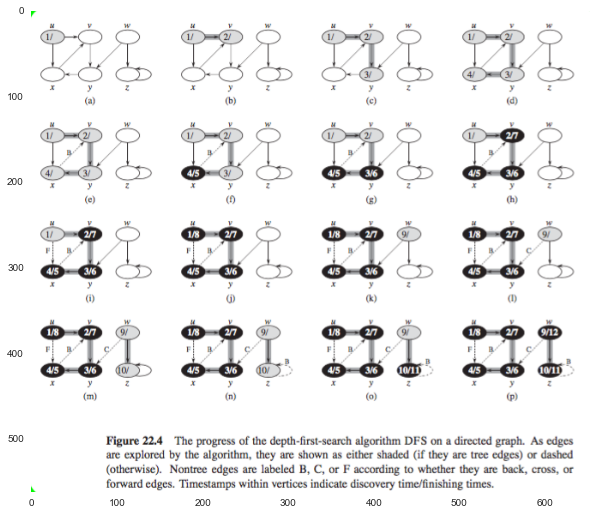##### properties¶
1. the predecessor subgraph $G_{\pi}$ does indeed form a forest of trees.

2. discovery and finishing times have parenthesis structure.

3. classification of edges:

• Tree edges edges in the depth-first forest $G_{\pi}$. WHITE
• Back edges edges $(u,v)$ connecting a vertex $u$ to an ancestor $v$ in a depth-first tree. GRAY
• Forward edges those nontree deges $(u,v)$ connecting a vertex $u$ to a descendant $v$ in a depth-first tree. BLACK
• Cross edges all other edges BLACK
In :
plt.figure(figsize=(10,20))

Out:
<matplotlib.image.AxesImage at 0x10cc12c18>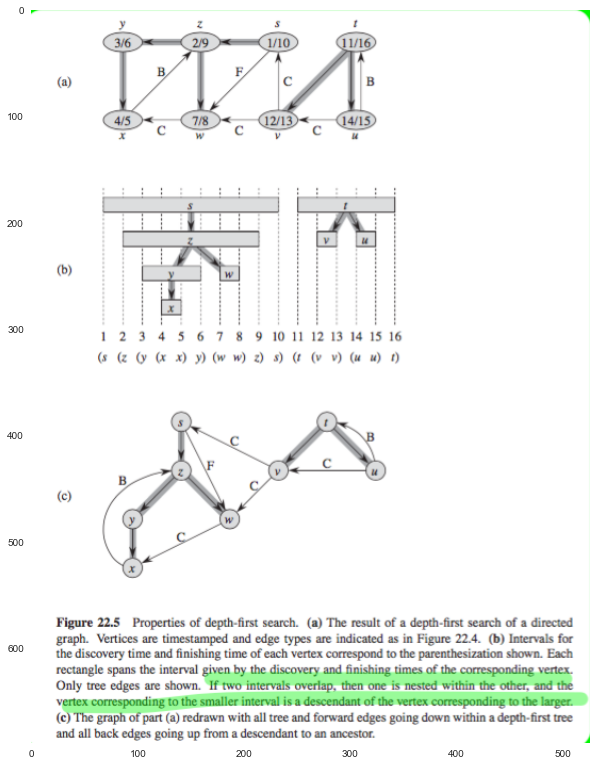In :
# Exercises


### 22.4 Topological sort¶

used for directed acyclic graph.

A topological sort of a dag $G = (V, E)$ is a linear ordering of all its vertices such that if $G$ contains an edge $(u,v)$, then $u$ appears before $v$ in the ordering.

Many applications use directed acyclic graphs to indicate precedences among events.

In :
plt.imshow(plt.imread('./res/tolo.png'))

Out:
<matplotlib.image.AxesImage at 0x1095ffc18>